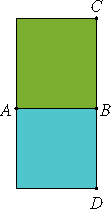# Proposition 24

The rectangle contained by medial straight lines commensurable in length is medial.

Let the rectangle AC be contained by the medial straight lines AB and BC which are commensurable in length.I say that AC is medial.

Describe the square AD on AB. Then AD is medial.

And, since AB is commensurable in length with BC, while AB equals BD, therefore DB is commensurable in length with BC, so that DA is commensurable with AC.

X.23,Cor.

But DA is medial, therefore AC is also medial.

Therefore, the rectangle contained by medial straight lines commensurable in length is medial.

Q.E.D.

## Guide

Medial lines were defined in Proposition X.21, but medial rectangles have not been defined. The meaning of the term medial rectangle must be interpreted by the way it is used in the proof of this proposition.

The square AD, which is the square on the medial line AB, is called a medial square, and since the rectangle AC is equal to the square AD, it is also proclaimed to be medial. Thus, a medial rectangle is one equal to the square on a medial line.

In this proposition, we have two medial numbers, a and b, such that a/b is a rational number. The conclusion is that c = √(ab) is a medial number. That is so since c2 = ab = b2(a/b), which is an irrational number, while c4 = a2b2 = b4(a/b)2, which is a rational number.

This proposition is not used in the rest of the Elements.